# Elementary flow

(diff) ← Older revision | Latest revision (diff) | Newer revision → (diff)
Jump to: navigation, search

A random sequence of moments of time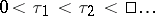at which the events of a flow of events take place (e.g. a flow of incoming calls at a telephone station), and for which the differencessatisfy the condition of independence and have the same exponential distribution. An elementary flow with distribution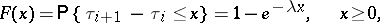(*)

is a particular case of a renewal process (cf. Renewal theory). To an elementary flow is related the Poisson process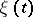equal to the number of events of the flow in the time interval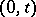. An elementary flow and its related Poisson process satisfy the following conditions.

Stationarity. For any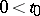,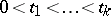the distribution of the random variable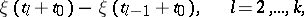does not depend on.

Orderliness. The probability of occurrence of two or more events of the flow in the intervalis equal to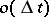as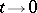.

Lack of memory. Forthe random variables,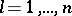, are independent.

It turns out that in these circumstances and under the condition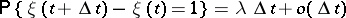the flow is elementary with exponential distribution (*).

How to Cite This Entry:
Elementary flow. Encyclopedia of Mathematics. URL: http://encyclopediaofmath.org/index.php?title=Elementary_flow&oldid=18634
This article was adapted from an original article by B.A. Sevast'yanov (originator), which appeared in Encyclopedia of Mathematics - ISBN 1402006098. See original article